# Hands-on Digital transmission with your soundcard¶

## 05 - Symbol Timing Recovery¶

In the previous notebook we have obtained a clear eye diagram. However, the eye opening was not centered and hence, sampling in center of the diagram would result in bit errors. In this notebook, we employ the Gardner algorithm for recovering the symbol timing such that we will be able to sample the eye diagram at the correct points in time.

The Gardner algorithm evaluates the eye diagram at twice the symbol rate. From these measurements, it calculates a metric to adjust the timing of the sampling step by step. Running the Gardner algorithm in a closed control loop always keeps track of the current symbol timing and hence will adjust timing to always sample at the correct timing instance.

Let's first import the standard modules:

In :
import matplotlib
import matplotlib.pyplot as plt
%matplotlib notebook

import numpy as np
import sys; sys.path.append('..')

In :
# Source code has been redacted in online version
# Omitting 4 lines of source code

In :
%matplotlib notebook

In :
# Source code has been redacted in online version
# Omitting 5 lines of source code


Then, let's again define our transmit signal generator as usual:

In :
# Source code has been redacted in online version
# Omitting 23 lines of source code


Let's again have a look at a part of the transmit signal:

In :
env = Environment(samplerate=44100)
transmitter = TransmitSignal(env, 'rc', 1/441)

signal = transmitter._generateSignal()
t = np.arange(len(signal)) / 44100 * 441
plt.figure(figsize=(8,3))
plt.plot(t, signal)
plt.grid(True); plt.xlim((10, 20)); plt.xlabel('t/Ts'); plt.ylabel('x(t)'); plt.tight_layout();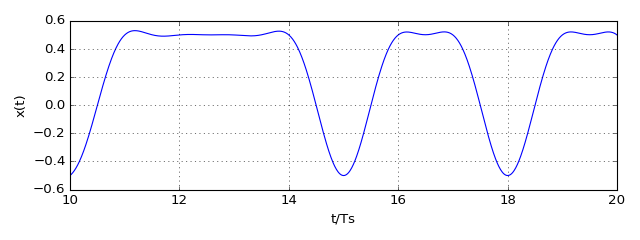Apparently, between the symbols there are sign changes, when adjacently transmitted bits differ. Moreover, the zero-crossing happens exactly in the middle between two symbols. This property is exploited by the Gardner algorithm. It samples the signal at twice the sampling rate and evaluates three adjacent samples.

Let's illustrate the Gardner algorithm with some code. Assume we constantly transmit flipping bits. In this case, we can approximate the transmit signal with a sine wave:

In :
plt.close('all'); plt.figure(figsize=(8,3))
samplerate = 44100
Ts = 1/441; Ns = samplerate * Ts
t = np.arange(4*Ns)/samplerate
x = np.cos(np.pi*t/Ts);
plt.plot(t/Ts, x); plt.grid(True); plt.xlabel('t/Ts'); plt.ylabel('x(t)'); plt.tight_layout();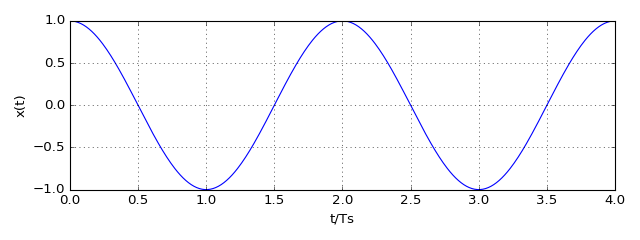Let's sample this signal at twice the symbol rate. The red dots indicate the assumption of the correct symbol timing, the blue dots indicate the sample between two symbol times. The Gardner metric $m[n]$ is given by

$$m[n] = (x[n] - x[n-2])x[n-1]$$

In the following code, this metric is calculated:

In :
# Source code has been redacted in online version
# Omitting 26 lines of source code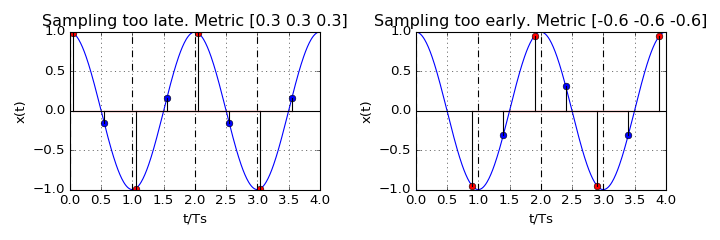As you can see, the sign of the metric indicates if we are sampling too early (metric is negative) or sampling too late (metric is positive). Moreover, the magnitude of the metric relates to the magnitude of the sampling offset.

This Gardner algorithm is used in a closed control loop fashion in the component. Let's use this implementation in our signal transmission chain to estimate the timing offset of the eye diagram. In addition, plot the estimated timing offset over the time and the eye diagram with no timing correction.

In :
# Source code has been redacted in online version
# Omitting 29 lines of source codeIn :
from audioComms.components import TimingRecovery
def runTransmission(Fc, channelFunc):
samplerate = 44100
env = Environment(samplerate=samplerate)

Ts = 1/(441)  # symbol duration
Ns = Ts * samplerate
t = np.arange(-4*Ts, 4*Ts, 1/samplerate)
transmitter = TransmitSignal(env, 'rc', Ts)
upconversion = Upconversion(env, Fc=Fc)

channel = channelFunc(env)

downconversion = Downconversion(env, Fc=Fc, B=4/Ts, removeCarrier=True)
timingRecovery = TimingRecovery(env, Ns)
fig = env.figure(figsize=(8,6))
plotEye = PlotEyeDiagram(env, Ts, axes=fig.add_subplot(221), title='Timing corrected eye diagram')
plotEyeUncorrected = PlotEyeDiagram(env, Ts, axes=fig.add_subplot(222), title='No timing correction')
plotWaveform = PlotWaveform(env, windowDuration=0.1, axes=fig.add_subplot(212), ylim=(-10-Ns, Ns+10), title='Estimated timing offset')

transmitter.transmitsTo(upconversion)
upconversion.transmitsTo(channel)
channel.transmitsTo(downconversion)
downconversion.transmitsTo(timingRecovery)
downconversion.transmitsTo(plotEyeUncorrected)
timingRecovery.transmitsTo(plotEye)
timingRecovery.transmitsTo(plotWaveform, stream='offset')

plt.tight_layout()
env.run()


First, let's run it in the simulated channel:

In :
runTransmission(18000, lambda env: SimulatedChannel(env, channelEffect=HighpassChannelEffect(gain=0.2)))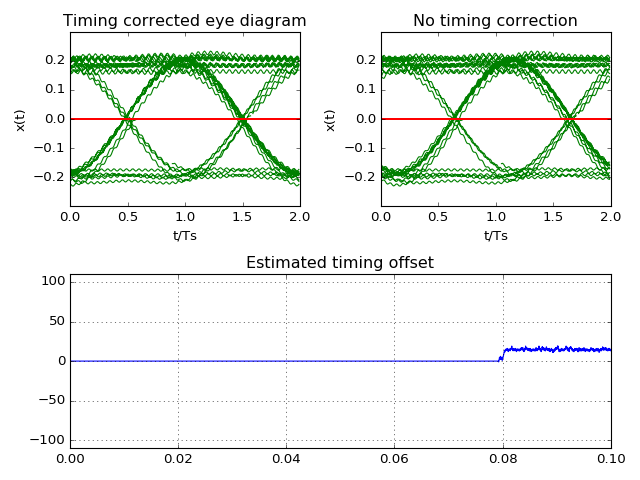Stop received from external


Nice! The eye opening is centered such that the sampling is correctly happening at the largest eye opening. Additionally, the estimated timing offset quickly converges to a constant value. Out of curiosity, let's define a channel effect that adds an additional random timing offset once in a while, by omitting some samples:

In :
from audioComms.channels import SimulatedChannelEffect
class SkipSamplesChannelEffect(SimulatedChannelEffect):
def response(self, data):
if np.random.rand() >= 0.99:
return data[15:]
elif np.random.rand() >= 0.99:
return np.hstack([data[:15], data])
else:
return data

In :
runTransmission(18000, lambda env: SimulatedChannel(env, SkipSamplesChannelEffect()))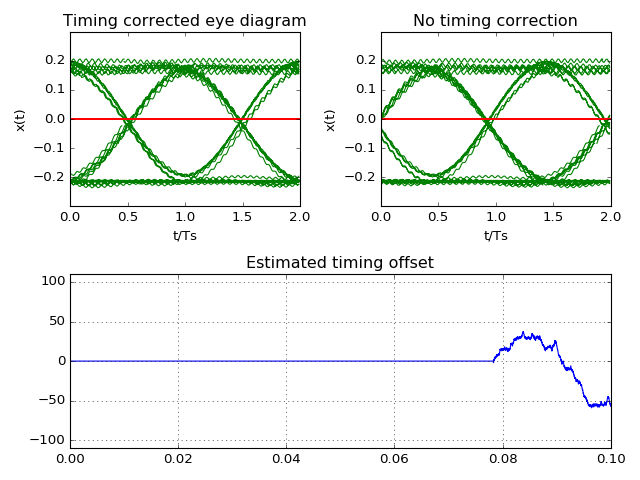Stop received from external


As you can see, the timing recovery reliably finds the correct eye opening, even if sometimes the correct timing moves. In these cases, the eye diagram shortly diverges, but quickly recovers.

Eventually, let's run our system in the real audio channel:

In :
runTransmission(18000, AudioChannel)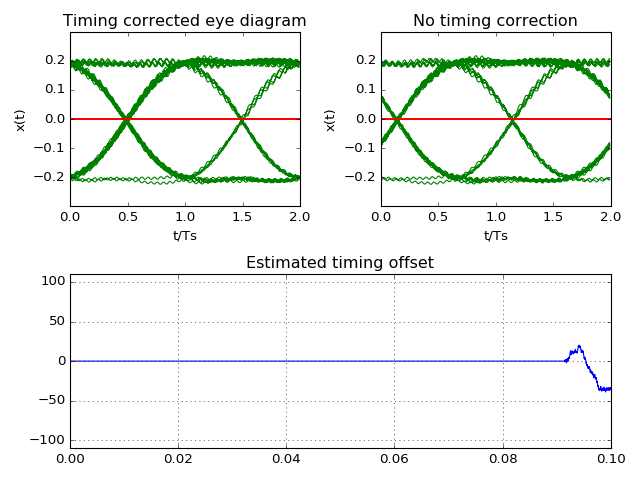Stop received from external


### Summary and Outlook¶

Now, that the eye diagram is centered, we can detect all the transmitted bits. So, we are ready to transmit real data, right? Well, not exactly.

Imagine sampling the eye diagram: it would generate a continuous stream of 0 and 1. However, with just a bit stream one does not know, what the data means. As an example problem, imagine the data represents bytes, i.e. collections of 8 subsequent bits are combined to one byte. How should you know, which bit is the MSB and which is the LSB? Naively, one could tell the first bit is the LSB, the 8th bit is the MSB and the 9th bit is the LSB of the next byte. However, what happens, if the first bit was not detected? How would you know what the first bit is? Then, the whole system would mix up the bytes. Hence, our system needs to have some structure within the data, in particular it has to describe the beginning of what is called a frame.

In the following notebook, we will introduce the most basic channel coding and interleaving to combat bit errors that occur during the transmission.